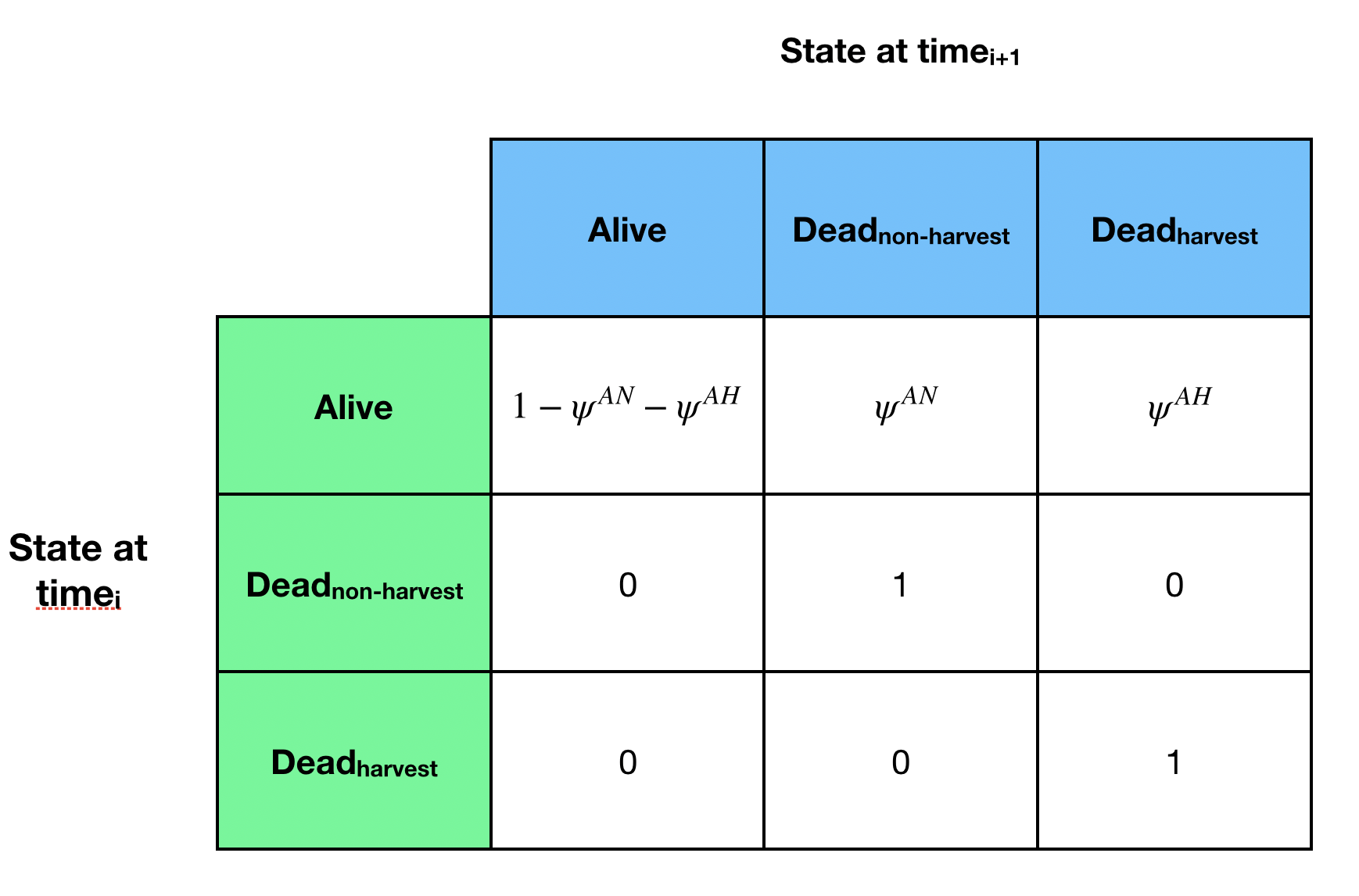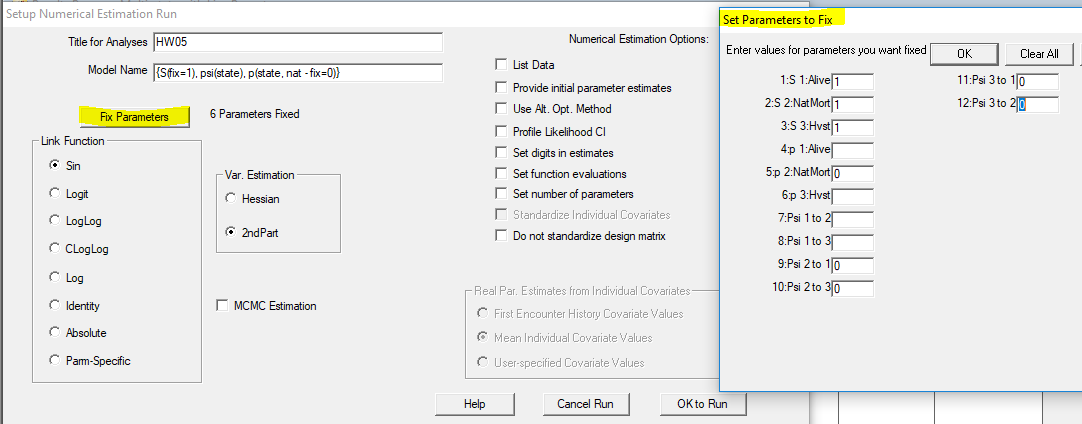For your homework for this week, please complete the following by the beginning of class next Tuesday.

Data set and analysis goals

This assignment will give you some experience with one of the many interesting ways in which multistate models can be used. The file “hw05.inp” contains information from a simulated multistate study conducted annually over 6 years. There are 3 states in the study, which are stored using the numbers 1, 2, and 3. The states are: 1 = alive or A, 2 = non-harvest-related mortality or N, and 3 = harvested or H. Here, the transitions of interest are transitions from state 1 to 1 ($$\psi^{AA}$$), 1 to 2 ($$\psi^{AN}$$), and 1 to 3 ($$\psi^{AH}$$). The values for other transitions are known and appear as shown below, e.g., $$\psi^{NN} = 1$$ as you can’t come back alive or switch among mortality causes. Because survival is being modeled with the transition $$\psi^{AA}$$, you will fix $$S=1$$ in the modeling. The live recaptures were collected with a method that provides decent, but by no means perfect, detection rates for individuals that remain alive. Animals that died due causes other than legal harvest were unobservable, i.e., $$p_N = 0$$. The study used an effective system for obtaining information on harvested individuals such that detection of individuals that were harvested was quite high but not perfect. Once an individual is harvested, i.e., has a 3 in its encounter history, the animal is removed from the study. This is accomplished by setting all subsequent values in the encounter history to 0 and assigning the individual a frequency of -1.Setting up the problem in Program MARK

Set the problem up in MARK by choosing the appropriate data type and inputting that you have data for 1 group and 0 individual covariates and that the input file has 3 states with values 1, 2 and 3. It’s helpful to give the 3 states short, meaningful names, e.g., “Alive”, “NatMort”, and “Hvst”. Note that MARK has default values of A, B, and C for the states, which you’ll need to replace with 1, 2, and 3.

1. How many PIMs are there for this problem?

2. How many columns are there in each of the PIMs?

3. For animals that are alive, what transitions do you have PIMs for? Can you change that? (Hint: check out MARK’s help manual that’s included in the program and search for “Multi-State Models” and the topic, “Transition Probabilities” under that topic.)

Models to evaluate

For the survival parameter, $$S$$, set the values in the PIMs for all 3 states to 1: remember you won’t use this parameter to estimate anything (see more below). For detection, you know that $$p$$ varied by state but wonder if $$p^{A}$$ is best modeled as constant or time-varying, i.e., $$p^A_.$$ and $$p^A_t$$. You are quite confident that $$p^H$$ was constant and know that $$p^{N} = 0$$, so you only need to evaluate 1 structure for each of those. You should run 2 model structures for the probabilities of dying: 1 that lets the probabilities vary by year and another that holds them constant during the study. That is, one model with $$\psi^{AN}_t$$ and $$\psi^{AH}_t$$ and a second model with $$\psi^{AN}_.$$ and $$\psi^{AH}_.$$. Each of the other transition PIMS can be filled in with 1 number as they’ll always be forced (fixed) to have a real parameter value of either 0 (for the impossible transitions, e.g., coming back to life) or 1 (for the only possible transition, i.e., stay dead). Thus, you’ll evaluate 4 models: 2 structures for $$p$$ and 2 structures for the probabilities of transitioning from the live state to one of the dead states (time-varying or constant).

By this point in the course, you’re probably getting pretty efficient at numbering PIMs in various ways. You might find it easiest to use the PIM chart for this set of exercises but do it however you wish.

For each of the models you run, you’ll need to use the “Fix Parameters” option on the “Setup Numerical Estimation Run” menu that appears after you hit “Run” for a given model. Fix all of the survival parameters to be 1, fix $$p^{N} = 0$$, and fix each of the impossible transitions to be 0. Note: the default PIMs obtain $$\psi^{NN}$$ and $$\psi^{HH}$$ by subtraction, so they will be forced to be 1 if you fix $$\psi^{NA}$$, $$\psi^{NH}$$, $$\psi^{HA}$$, and $$\psi^{HN}$$ all equal to 0. The graphic below shows a version of appropriate parameter fixing for a set of PIMs numbered by group and state but without any time variation. Realize that you are telling MARK what value you want each of those real parameters to be; those left blank are estimated.1. Provide an AIC table that is ordered by AICc (lowest at the top).

2. Based on the AIC table in the results browser, which model structure(s) was(were) most supported by the data and what general statements can you make about the key parameters you estimated?

3. For the top model, what are the estimated values for $$\psi^{AN}$$ and $$\psi^{AH}$$ and based on those, what is your estimate of $$\psi^{AA}$$?

4. What did you learn about detection of live and harvested animals?

5. For question 6, you provided an estimate of $$\psi^{AA}$$. You should, of course, also provide a measure of uncertainty for $$\psi^{AA}$$. There are a few ways to obtain that. First, retrieve your top model (highlight the model and use the “Retrieve” menu to retrieve it). Next, use the PIM menu to select “Change PIM Definition” and then change which PIM to obtain by subtraction. Specifically, choose to obtain Psi 1 to 2 or Psi 1 to 3 by subtraction. (Whenever you run a model and have previously fixed parameters, it’s a good idea to click on the “Fix Parameters” button to be sure the fixed parameter values are still as you want them.) Re-run your top model using the “Run” menu. Does the estimate for $$\psi^{AA}$$ match what you obtained in answering question 6? What is the standard error?

6. Another way of obtaining $$\widehat{SE}(\hat\psi^{AA})$$ is with the delta method, which is described in detail in Appendix B of CW. For this problem, you are working with the sum of $$\psi^{AN}$$ and $$\psi^{AH}$$ in the equation $$1 - (\psi^{AN}+\psi^{AH})$$ and so need to calculate the variance of a sum of 2 estimated quantities that also covary because they were estimated from the same data and model. The variance of the sum is $$var(\hat\psi^{AN})+var(\hat\psi^{AH})+ 2 \cdot cov({\hat\psi^{AN},\hat\psi^{AH})}$$. Think about the sum and how uncertainty about it arises. The greater the variances of the component pieces are, the less certain you are of the sum. But, the covariance also comes into play. If the 2 negatively covary, when one is higher, the other is lower such that the changes are offsetting to some degree, i.e., the sum is stabilized by the negative covariance. If, instead, they positively covary, the changes magnify changes in the sum to some degree. The equation used here captures that. Select the Output menu and choose “Specific Model Output”, “Variance-Covariance Matrices”, “Real Estimates”, and “Clipboard”. Then open an blank worksheet in Excel and paste the contents of the clipboard into Excel. Find the 2 rows and columns that correspond to $$\psi^{AN}$$ and $$\psi^{AH}$$, which is based on what rows of the design matrix they are on, which is determined by what PIM number corresponded to each of them. Sum those 4 values and take the square root: does that value match what you obtained in question 11 for $$\widehat{SE}(\hat\psi^{AA})$$?

7. Spend a bit of time with Lebreton et al. 2009 to see some of the many and interesting ways that multistate models have been used. Also, you could review some of the articles that cite this paper to learn of innovations related to this topic since 2009.